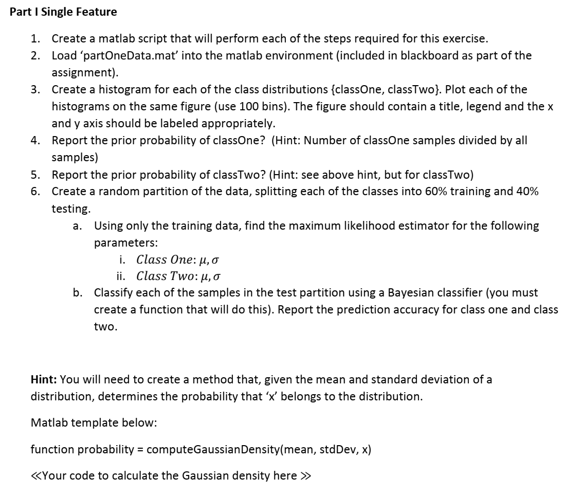# Create A Program to Create Pattern Recognition in Matlab Assignment Solution.

## Instructions

Objective
Write a program to create pattern recognition in matlab language.

## Requirements and SpecificationsSource Code

COMPUTE GAUSSIAN DENSITY

function probability = computeGaussianDensity(mean, stdDev, x)     probability = 1/(stdDev*sqrt(2*pi)) *exp(-(x-mean)^2 /(2*stdDev^2)); end 

QUESTION 1

clc, clear all, close all %% Part 2 load partOneData.mat N1 = length(classOne); % number of samples for class one N2 = length(classTwo); % "" "" "" class two N = N1+N2; %% Part 3 figure histogram(classOne, 100) hold on histogram(classTwo, 100) xlabel('No. Sample') ylabel('Value') title('Histogram for Class One and Class Two') grid on legend('class one', 'class two') %% Part 4 % Probability of class one prob_one = N1/N; fprintf("The probability for class one is: %.2f%%\n", prob_one*100); %% Part 5 prob_two = N2/N; fprintf("The probability for class two is: %.2f%%\n", prob_two*100); %% Part 6 test_size = 0.4; % percent of test training_size = 1-test_size; n_training = round(training_size*N1); % Shuffle data classOne = classOne(randperm(N1)); classTwo = classTwo(randperm(N2)); % Split into train and test train1 = classOne(1:n_training); train2 = classTwo(1:n_training); test1 = classOne(n_training+1:end); test2 = classTwo(n_training+1:end); %% part a) mu1 = mean(train1); sigma1 = std(train1); mu2 = mean(train2); sigma2 = std(train2); %% Part b) % We add a new column to each test vector to specify its label (! or 2) test1 = [test1', ones(length(test1),1)]; test2 = [test2', 2*ones(length(test2),1)]; % We combine both test datasets into one single matrix test = [test1;test2]; % Calculate the probabilities that a sample belongs to one class and label % them labels = []; for i = 1:length(test)     x = test(i,1);     label = test(i,2);     % Probabilities     prob = [computeGaussianDensity(mu1, sigma1, x)*prob_one, computeGaussianDensity(mu2, sigma2, x)*prob_two];     % Pik highest probability and label the sample based on that     [max_prob, y] = max(prob);     % Add label     labels = [labels;y]; end % Now, compute accuracy y = test(:,2); y_pred = labels; accuracy = sum(y==y_pred)/size(test,1); fprintf("The accuracy of the Bayesian classifier is: %.2f%%\n", accuracy*100); 

QUESTION 2

clc, clear all, close all %% Part 2 load partTwoData.mat N1 = size(classOne,1); % number of samples for class one N2 = size(classTwo,1); % "" "" "" class two N = N1+N2; %% Part 3 % Probability of class one prob_one = N1/N; fprintf("The probability for class one is: %.2f%%\n", prob_one*100); %% Part 4 prob_two = N2/N; fprintf("The probability for class two is: %.2f%%\n", prob_two*100); %% Part 5 test_size = 0.4; % percent of test training_size = 1-test_size; n_training = round(training_size*N1); % Shuffle data classOne = classOne(randperm(N1),:); classTwo = classTwo(randperm(N2),:); % Split into train and test train1 = classOne(1:n_training,:); train2 = classTwo(1:n_training,:); test1 = classOne(n_training+1:end,:); test2 = classTwo(n_training+1:end,:); %% part a) mu1 = mean(train1); E1 = corr(train1); mu2 = mean(train2); E2 = corr(train2); %% Part b) % We add a new column to each test vector to specify its label (! or 2) test1 = [test1, ones(length(test1),1)]; test2 = [test2, 2*ones(length(test2),1)]; % We combine both test datasets into one single matrix test = [test1;test2]; % Calculate the probabilities that a sample belongs to one class and label % them labels = []; for i = 1:length(test)     x = test(i,1:2);     label = test(i,3);     % Probabilities     prob = [computeGaussianDensityMultivariate(mu1, E1, x)*prob_one, computeGaussianDensityMultivariate(mu2, E2, x)*prob_two];     % Pik highest probability and label the sample based on that     [max_prob, y] = max(prob);     % Add label     labels = [labels;y]; end % Now, compute accuracy y = test(:,3); y_pred = labels; accuracy = sum(y==y_pred)/size(test,1); fprintf("The accuracy of the Bayesian classifier is: %.2f%%\n", accuracy*100);